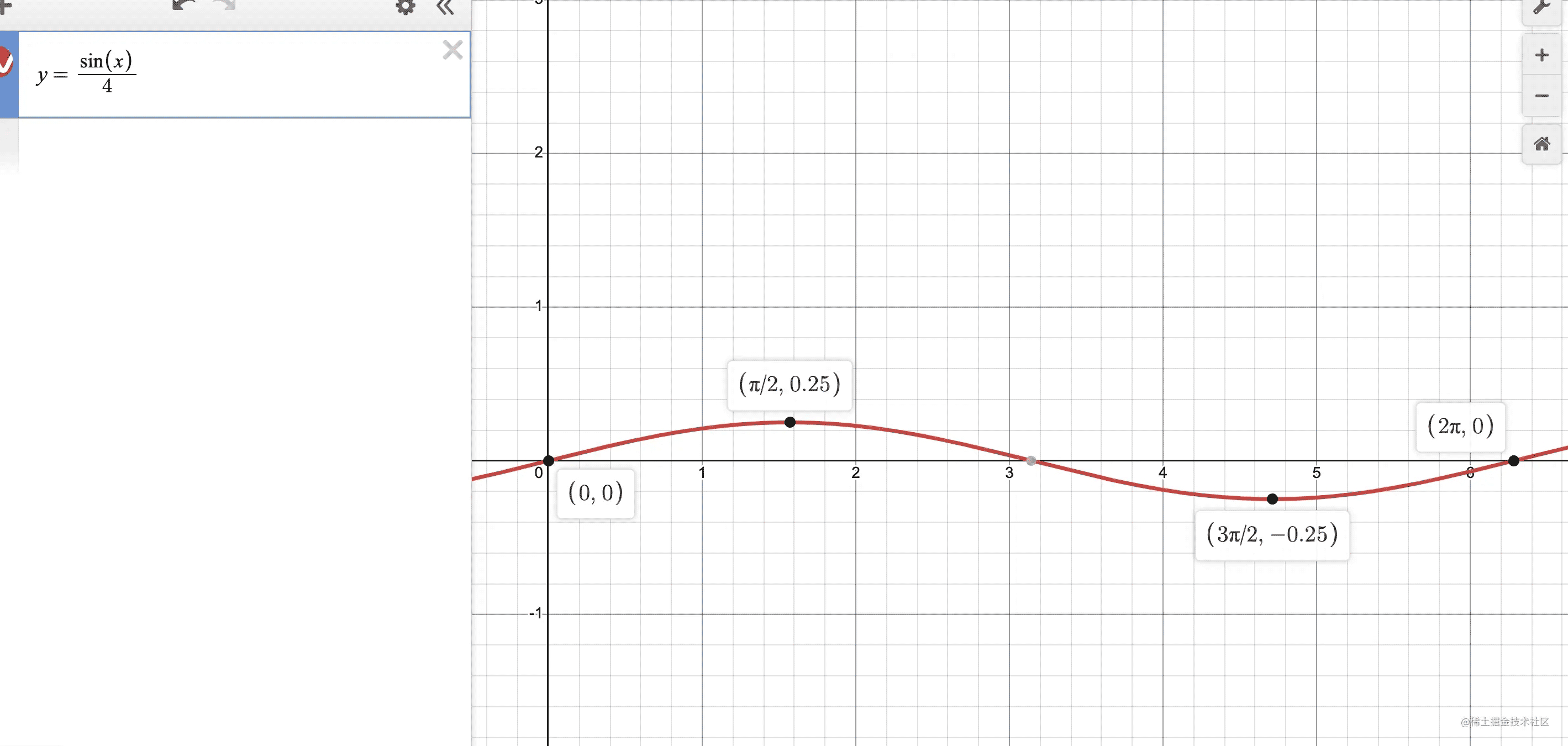﻿ js基于div丝滑实现贝塞尔曲线_JavaScript_脚本之家

# js基于div丝滑实现贝塞尔曲线

更新时间：2022年09月21日 09:37:46   作者：_小九

## 引言## 实现

### 布局

```<template>
<div class="container">
<div class="content">
<span class="circle" v-for="(item,index) in list" :key="index"></span>
</div>
</div>
</template>
```

### Math.sin() 和 Math.cos()

Math.sin(x)      x 的正玄值。返回值在 -1.0 到 1.0 之间；

Math.cos(x)    x 的余弦值。返回的是 -1.0 到 1.0 之间；sin(∠A) = 对边比斜边（a / c）
cos(∠A) = 临边比斜边 （b / c）

### 正弦曲线### 余弦曲线• 在曲线中 y = cos(x)
• 在曲线中，曲线的宽度是
• 在曲线中，曲线的高度最高点到最低点是2
• 在我们的需求中，总宽度是400px
• 在我们需求中， 共有二十个圆圈，所以我们可以算出每个球的宽度平均是20px，所以坐标就是`(index+1)*20`
• 现在我们知道了很多信息，我们就可以计算出更多信息了

### 计算更多信息

```/* 400 / x = 2π / y, 我们的x是已知的，等下自己可以拿，这样拿到了曲线中实际的x坐标 */
const z = 400 x / 400 * Math.PI*2
```

```/* 这样就拿到了曲线中的y坐标 */
y = Math.sin(z)
```

```/* 真实宽度400/曲线宽度2π = 真实高度y/曲线中的y 通过对比得到真实的y点 */
Y = Math.sin(z) * 400 / Math.PI * 2 / 2
```### 完善剩余### 完成跑马灯制作

```createActiveIndex(len = 6){
return Array.from({length:len}, (v,k) => k)
},
```

```changeIndex(){
this.activeIndex = this.activeIndex.map( item => item === this.list.length - 1 ? 0 : item + 1)
},
```## 贝塞尔曲线

### 简单封装一下方法

js中π就是Math.PI

```function getCoordinate(width, count,  mag = 1){
/* 通过总宽和个数计算出一个单个的宽 */
const singleWidth = width / count
/* 通过物理宽度/曲线周长计算出比率 */
const ratio = 400 / Math.PI*2
/* 上面实例代码我们是动态一次计算一个，而现在是方法，我们应该一次去拿到所有，所以我们返回一个数组对象记录xy */
let result = new Array(count).fill({})
/* 遍历总长度的dom个数，在数组中填充宽高 */
result = result.map( (item,index) => {
/* x的坐标 */
const x = (index + 1) * singleWidth
/* 定义变量z计算曲线中x的坐标 */
const z = x / width * Math.PI*2
/* 计算出真实的y的坐标 */
let y = Math.sin(z) / 4  * 400 / Math.PI * 2 / 2
/* y还需要通过倍率改变曲线，得到最终我们想要的y */
y = y / mag
/* 写入数组对象中 */
return {x, y}
})
return result;
} ```

## 完整示例

### style

```.container {
display: flex;
flex-direction: column;
justify-content: center;
align-items: center;
height: 100vh;
background-color: #000;
}
.content{
display: flex;
justify-content: space-between;
align-items: center;
width: 400px;
height: 50px;
}
.container .circle{
width: 15px;
height: 15px;
background-color: #befbf7;
}```

### SCript

```<template>
<div class="container">
<div class="content">
<span class="circle" v-for="(item,index) in list" :key="index" :style="{transform: `translateY(\${calcY(index)}px)`,backgroundColor: getCurrentBgColor(index)}"></span>
</div>
<div class="content" style="margin-top: 50px">
<span class="circle" v-for="(item,index) in list" :key="index" :style="{transform: `translateY(\${calcY(index)}px)`,backgroundColor: getRandomBgColor(index)}"></span>
</div>
</div>
</template>
<script>
export default {
data() {
return {
list: [], //定义总长度
activeIndex: [], // 运动中的球的颜色
interval: 300, //运动速度
colors: { // 定义轨道颜色和高亮颜色
active: '#2b88ff',
basic: '#b6f3f7'
},
cache: []
};
},
methods: {
init() {
this.list = new Array(20).fill(0)
this.start()
},
getCurrentBgColor(index){
return this.activeIndex.includes(index) ? '#6e9cae' : this.getRandomColor()
},
getRandomBgColor(index){
const color = this.activeIndex.includes(index) ? 'active' : 'basic'
return this.colors[color]
},
start(){
this.activeIndex = this.createActiveIndex()
setInterval(() => this.changeIndex(), this.interval)
},
changeIndex(){
this.activeIndex = this.activeIndex.map( item => item === this.list.length - 1 ? 0 : item + 1)
},
/* 生成需要动的球的个数 */
createActiveIndex(len = 6){
return Array.from({length:len}, (v,k) => k)
},
getRandomColor(){
return `#\${Math.floor(Math.random() * 0xffffff) .toString(16)}`;
},
getCoordinate(width, count,  mag = 1){
/* 通过总宽和个数计算出一个单个的宽 */
const singleWidth = width / count
/* 通过物理宽度/曲线周长计算出比率 */
const ratio = 400 / Math.PI*2
/* 上面实例代码我们是动态一次计算一个，而现在是方法，我们应该一次去拿到所有，所以我们返回一个数组对象记录xy */
let result = new Array(count).fill({})
/* 遍历总长度的dom个数，在数组中填充宽高 */
result = result.map( (item,index) => {
/* x的坐标 */
const x = (index + 1) * singleWidth
/* 定义变量z计算曲线中x的坐标 */
const z = x / width * Math.PI*2
/* 计算出真实的y的坐标 */
let y = Math.sin(z) / 4  * 400 / Math.PI * 2 / 2
/* y还需要通过倍率改变曲线，得到最终我们想要的y */
y = y / mag
/* 写入数组对象中 */
return {x, y}
})
return result;
}
},
created(){
this.cache = this.getCoordinate(400, 20, 1)
this.init()
},
computed:{
calcY(){
return (index) => {
/* 使用封装的方法计算 */
// return this.cache[index].y
const x = (index + 1) * 20
const z = x / 400 * Math.PI*2
const y = Math.sin(z) * 400 / Math.PI * 2 / 2 / 4
return y
}
}
}
};
</script>```